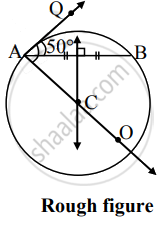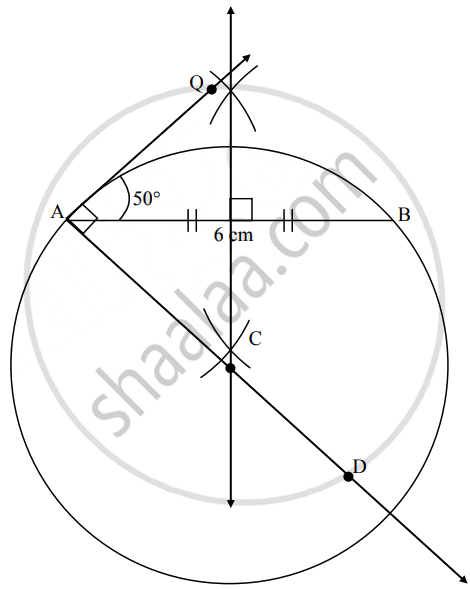# AB = 6 cm, ∠BAQ = 50°. Draw a circle passing through A and B so that AQ is the tangent to the circle - Geometry

Diagram

AB = 6 cm, ∠BAQ = 50°. Draw a circle passing through A and B so that AQ is the tangent to the circle

#### SolutionAnalysis: As shown in the figure,

C is the centre of the required circle.

∴ ∠QAC = 90°    ......[Tangent theorem]

∴ Centre of the circle must be on ray AC and it must be equidistant from point A and point B.

∴ The centre of the circle, i.e., point C, is the point of intersection of ray AC and perpendicular bisector of seg AB.Steps of construction:

1. Draw seg AB of 6 cm.
2. Draw ray AQ such that ∠BAQ = 50°
4. Draw perpendicular bisector of seg AB, intersecting ray AD at point C.
5. With centre C, draw a circle with radius AC.
Concept: Construction of a Tangent to the Circle at a Point on the Circle
Is there an error in this question or solution?

Share